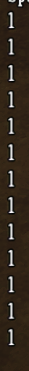## Recommended Posts

Is there any way to alter any of the code in constants?

##### Share on other sites

Yep. You alter it the same way you alter any other variable.

Examples:

```GLOBAL.ANCHOR_MIDDLE = 999 -- break all the UIs
GLOBAL.EQUIPSLOTS.LEG = "leg"```

Edited by penguin0616
•1
•1
##### Share on other sites

```local PIGTRADE_ONE = { PIGTRADE = 1 }

--print(GLOBAL.constants)

Sorry, so it there anything i'm doing wrong

```TECH =
{
NONE = TechTree.Create(),

SCIENCE_ONE = { SCIENCE = 1 },
SCIENCE_TWO = { SCIENCE = 2 },
SCIENCE_THREE = { SCIENCE = 3 },
-- Magic starts at level 2 so it's not teased from the start.

--bit of refrence```

print(GLOBAL.TECH.PIGTRADE_ONE) will always come as nil for some reason

##### Share on other sites

Since you are using table.insert, it would end up as:

```TECH =
{
NONE = TechTree.Create(),

SCIENCE_ONE = { SCIENCE = 1 },
SCIENCE_TWO = { SCIENCE = 2 },
SCIENCE_THREE = { SCIENCE = 3 },
 = { PIGTRADE = 1 },
 = { PIGTRADE = 2 },
}
```

Change

```table.insert(GLOBAL.TECH, PIGTRADE_ONE)

to

```GLOBAL.TECH.PIGTRADE_ONE = PIGTRADE_ONE

Which will leave you with

```TECH =
{
NONE = TechTree.Create(),

SCIENCE_ONE = { SCIENCE = 1 },
SCIENCE_TWO = { SCIENCE = 2 },
SCIENCE_THREE = { SCIENCE = 3 },
}```

•2
##### Share on other sitesbeautiful ones, thanks

•1